Courses

# Test: Congruence Of Triangles - 2

## 20 Questions MCQ Test Mathematics (Maths) Class 7 | Test: Congruence Of Triangles - 2

Description
This mock test of Test: Congruence Of Triangles - 2 for Class 7 helps you for every Class 7 entrance exam. This contains 20 Multiple Choice Questions for Class 7 Test: Congruence Of Triangles - 2 (mcq) to study with solutions a complete question bank. The solved questions answers in this Test: Congruence Of Triangles - 2 quiz give you a good mix of easy questions and tough questions. Class 7 students definitely take this Test: Congruence Of Triangles - 2 exercise for a better result in the exam. You can find other Test: Congruence Of Triangles - 2 extra questions, long questions & short questions for Class 7 on EduRev as well by searching above.
QUESTION: 1

### What comes next in the sequence: 2, 4, 10, 28, ___ ?

Solution:

The difference between 2 and 4 is 2

The difference between 4 and 10 is 6 (or 2 from previous x3)

The difference between 10 and 28 is 18 (or 6 from previous x 3)

The difference between 28 and 82 is is 54 (or 18 from previous x 3)

QUESTION: 2

### What is the side included between the angles A and B in ΔABC?

Solution:

Angle A and Angle B have a line as AB , since we have two vertices A and B which gives us the side AB.

QUESTION: 3

### In the below quadrilateral ABCD, AD = BC and ∠DAB = ∠CBA. If ΔABD ≌ ΔBAC. The relation between ∠ABD and ∠BAC is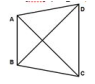Solution:

In ΔABD and ΔBAC,

∠DAB = ∠CBA (Given)

AB = BA (Common)

∴ ΔABD ≅ ΔBAC (By SAS congruence rule)

∴ BD = AC (By CPCT)

And, ∠ABD = ∠BAC (By CPCT)

QUESTION: 4

In the quadrilateral ABCD, AC = AD and AB bisect ∠A and ΔABC ≅ ΔABD. The relation between BC and BD is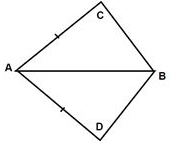Solution:
QUESTION: 5

In the below figure, AC and BD are equal perpendicular to line segment AB. If ?BOC ≅ ?AOD, then the relation between OC and OD is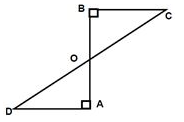Solution:
QUESTION: 6

In the below figure, AB = AC = CD. If ΔACD ≌ ΔABE then AD =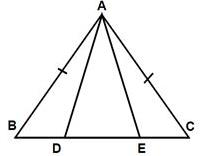Solution:

ΔACD ≌ ΔABE. Here angle A corresponds to Angle A, Angle C with Angle B and Angle D with Angle E, Similarly Side AC corresponds to AB, CD to BE and AD to AE. So AE is the answer.

QUESTION: 7

ΔABC is right triangle in which ∠A = 90° and AB = AC. The values of ∠B and ∠C will be

Solution:
QUESTION: 8

The measure of each angle of an equilateral triangle is:

Solution:
QUESTION: 9

If the vertical angle of a isosceles triangle is 40°, then measure of other two angles will be

Solution:
QUESTION: 10

If ∠A, ∠B and ∠C of ΔABC are equal, then triangle is

Solution:
QUESTION: 11

Which one of the following is the value of congruency?

Solution:

We have four congruence criterions namely, SSS,SAS,ASA,RHS. There is no such ASS or SSA criterions

QUESTION: 12

By which congruence rule following triangles are congruent ?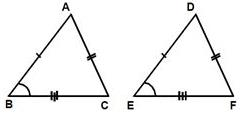Solution:

The SSS rule states that:

If three sides of one triangle are equal to three sides of another triangle, then the triangles are congruent.

QUESTION: 13

In the below figure, AC = BD and AD = BC. Which of the following statements is meaningfully written?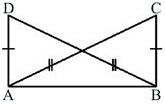Solution:

AC corresponds to BD and AD corresponds to BC. So while writing the name of the triangles the alphabets should be written according to the correspondence. So ΔABC ≅ ΔBAD

QUESTION: 14

Which is the longest side in the triangle ABC right angled at B?

Solution:
QUESTION: 15

Which is the longest side of a right triangle?

Solution:
QUESTION: 16

What is the angle included between the sides PN and PM of ΔMNP?

Solution:
QUESTION: 17

In triangles ABC and PQR, AB = 3.5 cm, BC = 7.1 cm, AC = 5 cm, PQ = 7.1 cm, QR = 5 cm and PR = 3.5 cm, then which of the following is true

Solution:
QUESTION: 18

A triangle in which all three sides are of equal lengths is called _________.

Solution:

Equilateral means having all sides equal .So Equilateral triangle has all of its 3 sides equal.

QUESTION: 19

In triangles ABC and DEF, AB = 7 cm, BC = 5 cm, ∠B = 50°, DE = 5 cm, EF = 7 cm, ∠E = 50°. By which congruence rule the triangles are congruent?

Solution:

Given: AB = EF = 7 cm,

BC = DE = 5 cm and

∠B =∠E = 50°

Also,

A↔F,B↔E,C↔D

By using SAS congruence rule, it can be written as

∆ABC ≅ ∆FED

QUESTION: 20

In the below figure, A B = AC and AD is the bisector of ∠BAC , then the relation between ∠B and ∠C is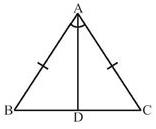Solution:

AB=AC(given)